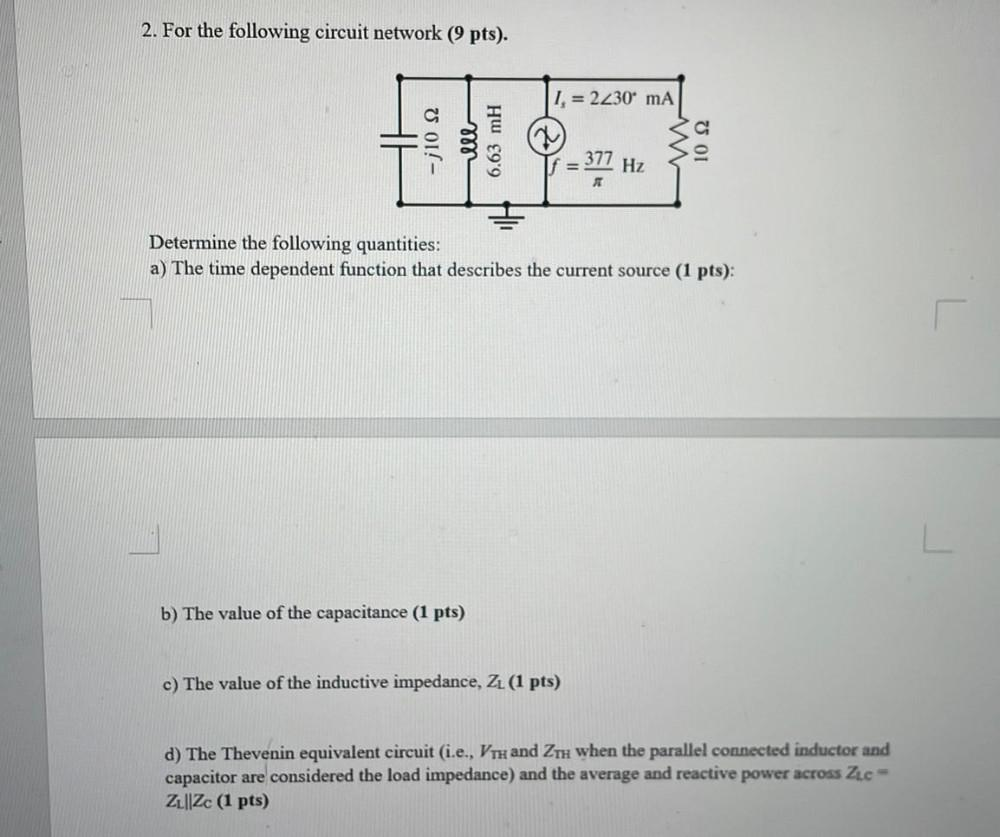Question:

# 2. For the following circuit network (9 pts). 1. = 2230 mA -210 S2 377 Hz Determine the following quantities: a) The time depend2. For the following circuit network (9 pts). 1. = 2230 mA -210 S2 377 Hz Determine the following quantities: a) The time dependent function that describes the current source (1 pts): b) The value of the capacitance (1 pts) c) The value of the inductive impedance, ZL (1 pts) d) The Thevenin equivalent circuit (i.e., VTH and ZTH when the parallel connected inductor and capacitor are considered the load impedance) and the average and reactive power across Zee Z1||Zc (1 pts)